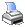﻿ MCL Reference > Null Values > Propagation of Null Values
 Navigation:  MCL Reference > Null Values > Propagation of Null ValuesWhenever a formula contains an item of null value, the formula always gives a result of .NULL. except that the null value is the argument of the function IsNull( ) or the first argument of NVL( ).

For example, if you write a formula:

14 - Column('Ave FP')

and the value of the item Column('Ave FP') is .NULL., the result yielded from the formula will be .NULL.

i.e. 14 - .NULL. = .NULL.